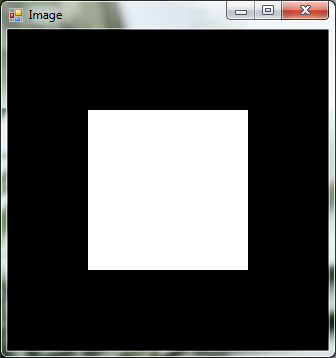﻿ MatrixToImage Class# MatrixToImage Class

Multidimensional array to Bitmap converter.Inheritance Hierarchy
SystemObject
Accord.Imaging.ConvertersMatrixToImage

Namespace:  Accord.Imaging.Converters
Assembly:  Accord.Imaging (in Accord.Imaging.dll) Version: 3.8.0Syntax
```public class MatrixToImage : IConverter<double[,], Bitmap>,
IConverter<double[,], UnmanagedImage>, IConverter<float[,], Bitmap>,
IConverter<float[,], UnmanagedImage>, IConverter<byte[,], Bitmap>,
IConverter<byte[,], UnmanagedImage>, IConverter<int[,], Bitmap>,
IConverter<int[,], UnmanagedImage>, IConverter<short[,], Bitmap>,
IConverter<short[,], UnmanagedImage>, IConverter<double[][], Bitmap>,
IConverter<double[][], UnmanagedImage>, IConverter<float[][], Bitmap>,
IConverter<float[][], UnmanagedImage>, IConverter<byte[][], Bitmap>,
IConverter<byte[][], UnmanagedImage>```

The MatrixToImage type exposes the following members.Constructors
NameDescriptionMatrixToImage
Initializes a new instance of the MatrixToImage class.MatrixToImage(Double, Double)
Initializes a new instance of the MatrixToImage class.
TopProperties
NameDescriptionFormat
Gets or sets the desired output format of the image.Max
Gets or sets the maximum double value in the double array associated with the brightest color.Min
Gets or sets the minimum double value in the double array associated with the darkest color.
TopMethods
NameDescriptionConvert(Byte, UnmanagedImage)
Converts an image from one representation to another.Convert(Byte, Bitmap)
Converts an image from one representation to another.Convert(Byte, UnmanagedImage)
Converts an image from one representation to another.Convert(Byte, Bitmap)
Converts an image from one representation to another.Convert(Double, UnmanagedImage)
Converts an image from one representation to another.Convert(Double, Bitmap)
Converts an image from one representation to another.Convert(Double, UnmanagedImage)
Converts an image from one representation to another.Convert(Double, Bitmap)
Converts an image from one representation to another.Convert(Int16, UnmanagedImage)
Converts an image from one representation to another.Convert(Int16, Bitmap)
Converts an image from one representation to another.Convert(Int16, UnmanagedImage)
Converts an image from one representation to another.Convert(Int16, Bitmap)
Converts an image from one representation to another.Convert(Int32, UnmanagedImage)
Converts an image from one representation to another.Convert(Int32, Bitmap)
Converts an image from one representation to another.Convert(Int32, UnmanagedImage)
Converts an image from one representation to another.Convert(Int32, Bitmap)
Converts an image from one representation to another.Convert(Single, UnmanagedImage)
Converts an image from one representation to another.Convert(Single, Bitmap)
Converts an image from one representation to another.Convert(Single, UnmanagedImage)
Converts an image from one representation to another.Convert(Single, Bitmap)
Converts an image from one representation to another.Equals
Determines whether the specified object is equal to the current object.
(Inherited from Object.)Finalize
Allows an object to try to free resources and perform other cleanup operations before it is reclaimed by garbage collection.
(Inherited from Object.)GetHashCode
Serves as the default hash function.
(Inherited from Object.)GetType
Gets the Type of the current instance.
(Inherited from Object.)MemberwiseClone
Creates a shallow copy of the current Object.
(Inherited from Object.)ToString
Returns a string that represents the current object.
(Inherited from Object.)
TopExtension Methods
NameDescriptionHasMethod
Checks whether an object implements a method with the given name.
(Defined by ExtensionMethods.)IsEqual
Compares two objects for equality, performing an elementwise comparison if the elements are vectors or matrices.
(Defined by Matrix.)To(Type)Overloaded.
Converts an object into another type, irrespective of whether the conversion can be done at compile time or not. This can be used to convert generic types to numeric types during runtime.
(Defined by ExtensionMethods.)ToTOverloaded.
Converts an object into another type, irrespective of whether the conversion can be done at compile time or not. This can be used to convert generic types to numeric types during runtime.
(Defined by ExtensionMethods.)
TopRemarks
This class can convert double and float multidimensional arrays (matrices) to Grayscale bitmaps. The color representation of the values contained in the matrices must be specified through the Min and Max properties of the class or class constructor.Examples

This example converts a multidimensional array of double-precision floating-point numbers with values from 0 to 1 into a grayscale image.

```// Create a matrix representation
// of a 4x4 image with a inner 2x2
// square drawn in the middle

double[,] pixels =
{
{ 0, 0, 0, 0 },
{ 0, 1, 1, 0 },
{ 0, 1, 1, 0 },
{ 0, 0, 0, 0 },
};

// Create the converter to convert the matrix to a image
MatrixToImage conv = new MatrixToImage(min: 0, max: 1);

// Declare an image and store the pixels on it
Bitmap image; conv.Convert(pixels, out image);

// Show the image on screen
image = new ResizeNearestNeighbor(320, 320).Apply(image);
ImageBox.Show(image, PictureBoxSizeMode.Zoom);```

The resulting image is shown below.See Also